7 Work and Kinetic Energy

# 7.1 Work

### Learning Objectives

By the end of this section, you will be able to:

• Represent the work done by any force
• Evaluate the work done for various forces

In physics, work is done on an object when energy is transferred to the object. In other words, work is done when a force acts on something that undergoes a displacement from one position to another. Forces can vary as a function of position, and displacements can be along various paths between two points. We first define the increment of work dW done by a force $\mathbf{\overset{\to }{F}}$ acting through an infinitesimal displacement $d\mathbf{\overset{\to }{r}}$ as the dot product of these two vectors:

$dW=\mathbf{\overset{\to }{F}}\cdot d\mathbf{\overset{\to }{r}}=|\mathbf{\overset{\to }{F}}||d\mathbf{\overset{\to }{r}}|\text{cos}\,\theta .$

Then, we can add up the contributions for infinitesimal displacements, along a path between two positions, to get the total work.

#### Work Done by a Force

The work done by a force is the integral of the force with respect to displacement along the path of the displacement:

${W}_{AB}=\underset{\text{path}\,AB}{\int }\mathbf{\overset{\to }{F}}\cdot d\mathbf{\overset{\to }{r}}.$

The vectors involved in the definition of the work done by a force acting on a particle are illustrated in Figure.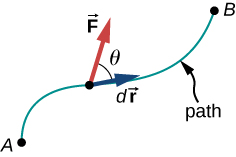Figure 7.2 Vectors used to define work. The force acting on a particle and its infinitesimal displacement are shown at one point along the path between A and B. The infinitesimal work is the dot product of these two vectors; the total work is the integral of the dot product along the path.

We choose to express the dot product in terms of the magnitudes of the vectors and the cosine of the angle between them, because the meaning of the dot product for work can be put into words more directly in terms of magnitudes and angles. We could equally well have expressed the dot product in terms of the various components introduced in Vectors. In two dimensions, these were the x– and y-components in Cartesian coordinates, or the r– and $\phi$-components in polar coordinates; in three dimensions, it was just x-, y-, and z-components. Which choice is more convenient depends on the situation. In words, you can express Figure for the work done by a force acting over a displacement as a product of one component acting parallel to the other component. From the properties of vectors, it doesn’t matter if you take the component of the force parallel to the displacement or the component of the displacement parallel to the force—you get the same result either way.

Recall that the magnitude of a force times the cosine of the angle the force makes with a given direction is the component of the force in the given direction. The components of a vector can be positive, negative, or zero, depending on whether the angle between the vector and the component-direction is between $0^\circ$ and $90^\circ$ or $90^\circ$ and $180^\circ$, or is equal to $90^\circ$. As a result, the work done by a force can be positive, negative, or zero, depending on whether the force is generally in the direction of the displacement, generally opposite to the displacement, or perpendicular to the displacement. The maximum work is done by a given force when it is along the direction of the displacement ($\text{cos}\,\theta =\pm1$), and zero work is done when the force is perpendicular to the displacement ($\text{cos}\,\theta =0$).

The units of work are units of force multiplied by units of length, which in the SI system is newtons times meters, $\text{N}\cdot \text{m.}$ This combination is called a joule, for historical reasons that we will mention later, and is abbreviated as J. In the English system, still used in the United States, the unit of force is the pound (lb) and the unit of distance is the foot (ft), so the unit of work is the foot-pound $(\text{ft}\cdot \text{lb})\text{.}$

### Work Done by Constant Forces and Contact Forces

The simplest work to evaluate is that done by a force that is constant in magnitude and direction. In this case, we can factor out the force; the remaining integral is just the total displacement, which only depends on the end points A and B, but not on the path between them:

${W}_{AB}=\mathbf{\overset{\to }{F}}\cdot {\int }_{A}^{B}d\mathbf{\overset{\to }{r}}=\mathbf{\overset{\to }{F}}\cdot ({\mathbf{\overset{\to }{r}}}_{B}-{\mathbf{\overset{\to }{r}}}_{A})=|\mathbf{\overset{\to }{F}}||{\mathbf{\overset{\to }{r}}}_{B}-{\mathbf{\overset{\to }{r}}}_{A}|\text{cos}\,\theta \enspace\text{(constant force).}$

We can also see this by writing out Figure in Cartesian coordinates and using the fact that the components of the force are constant:

$\begin{array}{cc}\hfill {W}_{AB}& =\underset{\text{path}\,AB}{\int }\mathbf{\overset{\to }{F}}\cdot d\mathbf{\overset{\to }{r}}=\underset{\text{path}\,AB}{\int }({F}_{x}dx+{F}_{y}dy+{F}_{z}dz)={F}_{x}{\int }_{A}^{B}dx+{F}_{y}{\int }_{A}^{B}dy+{F}_{z}{\int }_{A}^{B}dz\hfill \\ & ={F}_{x}({x}_{B}-{x}_{A})+{F}_{y}({y}_{B}-{y}_{A})+{F}_{z}({z}_{B}-{z}_{A})=\mathbf{\overset{\to }{F}}\cdot ({\mathbf{\overset{\to }{r}}}_{B}-{\mathbf{\overset{\to }{r}}}_{A}).\hfill \end{array}$

Figure(a) shows a person exerting a constant force $\mathbf{\overset{\to }{F}}$ along the handle of a lawn mower, which makes an angle $\theta$ with the horizontal. The horizontal displacement of the lawn mower, over which the force acts, is $\mathbf{\overset{\to }{d}}.$ The work done on the lawn mower is$W=\mathbf{\overset{\to }{F}}\cdot \mathbf{\overset{\to }{d}}=Fd\,\text{cos}\,\theta$, which the figure also illustrates as the horizontal component of the force times the magnitude of the displacement.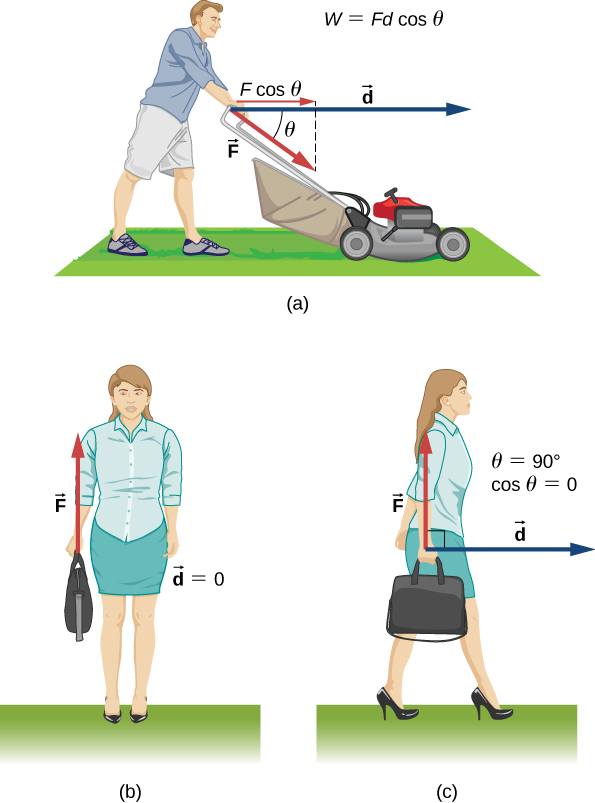Figure 7.3 Work done by a constant force. (a) A person pushes a lawn mower with a constant force. The component of the force parallel to the displacement is the work done, as shown in the equation in the figure. (b) A person holds a briefcase. No work is done because the displacement is zero. (c) The person in (b) walks horizontally while holding the briefcase. No work is done because $\text{cos}\,\theta$ is zero.

Figure(b) shows a person holding a briefcase. The person must exert an upward force, equal in magnitude to the weight of the briefcase, but this force does no work, because the displacement over which it acts is zero. So why do you eventually feel tired just holding the briefcase, if you’re not doing any work on it? The answer is that muscle fibers in your arm are contracting and doing work inside your arm, even though the force your muscles exert externally on the briefcase doesn’t do any work on it. (Part of the force you exert could also be tension in the bones and ligaments of your arm, but other muscles in your body would be doing work to maintain the position of your arm.)

In Figure(c), where the person in (b) is walking horizontally with constant speed, the work done by the person on the briefcase is still zero, but now because the angle between the force exerted and the displacement is $90^\circ$ ($\mathbf{\overset{\to }{F}}$ perpendicular to $\mathbf{\overset{\to }{d}}$) and $\text{cos}\,90^\circ=0$.

### Example

#### Calculating the Work You Do to Push a Lawn Mower

How much work is done on the lawn mower by the person in Figure(a) if he exerts a constant force of 75.0 N at an angle $35^\circ$ below the horizontal and pushes the mower 25.0 m on level ground?

#### Strategy

We can solve this problem by substituting the given values into the definition of work done on an object by a constant force, stated in the equation $W=Fd\,\text{cos}\,\theta$. The force, angle, and displacement are given, so that only the work W is unknown.

#### Solution

The equation for the work is

$W=Fd\,\text{cos}\,\theta .$

Substituting the known values gives

$W=(75.0\,\text{N})(25.0\,\text{m})\text{cos}(35.0^\circ)=1.54\times {10}^{3}\,\text{J}\text{.}$

#### Significance

Even though one and a half kilojoules may seem like a lot of work, we will see in Potential Energy and Conservation of Energy that it’s only about as much work as you could do by burning one sixth of a gram of fat.

When you mow the grass, other forces act on the lawn mower besides the force you exert—namely, the contact force of the ground and the gravitational force of Earth. Let’s consider the work done by these forces in general. For an object moving on a surface, the displacement $d\mathbf{\overset{\to }{r}}$ is tangent to the surface. The part of the contact force on the object that is perpendicular to the surface is the normal force $\mathbf{\overset{\to }{N}}.$ Since the cosine of the angle between the normal and the tangent to a surface is zero, we have

$d{W}_{\text{N}}=\mathbf{\overset{\to }{N}}\cdot d\mathbf{\overset{\to }{r}}=\mathbf{\overset{\to }{0}}.$

The normal force never does work under these circumstances. (Note that if the displacement $d\mathbf{\overset{\to }{r}}$ did have a relative component perpendicular to the surface, the object would either leave the surface or break through it, and there would no longer be any normal contact force. However, if the object is more than a particle, and has an internal structure, the normal contact force can do work on it, for example, by displacing it or deforming its shape. This will be mentioned in the next chapter.)

The part of the contact force on the object that is parallel to the surface is friction, $\mathbf{\overset{\to }{f}}.$ For this object sliding along the surface, kinetic friction ${\mathbf{\overset{\to }{f}}}_{\text{k}}$ is opposite to $d\mathbf{\overset{\to }{r}},$ relative to the surface, so the work done by kinetic friction is negative. If the magnitude of ${\mathbf{\overset{\to }{f}}}_{\text{k}}$ is constant (as it would be if all the other forces on the object were constant), then the work done by friction is

${W}_{\text{fr}}={\int }_{A}^{B}{\mathbf{\overset{\to }{f}}}_{k}\cdot d\mathbf{\overset{\to }{r}}=\text{−}{f}_{k}{\int }_{A}^{B}|dr|=\text{−}{f}_{k}|{l}_{AB}|,$

where $|{l}_{AB}|$ is the path length on the surface. (Note that, especially if the work done by a force is negative, people may refer to the work done against this force, where $d{W}_{\text{against}}=\text{−}d{W}_{\text{by}}$. The work done against a force may also be viewed as the work required to overcome this force, as in “How much work is required to overcome…?”) The force of static friction, however, can do positive or negative work. When you walk, the force of static friction exerted by the ground on your back foot accelerates you for part of each step. If you’re slowing down, the force of the ground on your front foot decelerates you. If you’re driving your car at the speed limit on a straight, level stretch of highway, the negative work done by kinetic friction of air resistance is balanced by the positive work done by the static friction of the road on the drive wheels. You can pull the rug out from under an object in such a way that it slides backward relative to the rug, but forward relative to the floor. In this case, kinetic friction exerted by the rug on the object could be in the same direction as the displacement of the object, relative to the floor, and do positive work. The bottom line is that you need to analyze each particular case to determine the work done by the forces, whether positive, negative or zero.

### Example

#### Moving a Couch

You decide to move your couch to a new position on your horizontal living room floor. The normal force on the couch is 1 kN and the coefficient of friction is 0.6. (a) You first push the couch 3 m parallel to a wall and then 1 m perpendicular to the wall (A to B in Figure). How much work is done by the frictional force? (b) You don’t like the new position, so you move the couch straight back to its original position (B to A in Figure). What was the total work done against friction moving the couch away from its original position and back again?

#### Strategy

The magnitude of the force of kinetic friction on the couch is constant, equal to the coefficient of friction times the normal force, ${f}_{K}={\mu }_{K}N$. Therefore, the work done by it is ${W}_{\text{fr}}=\text{−}{f}_{K}d$, where d is the path length traversed. The segments of the paths are the sides of a right triangle, so the path lengths are easily calculated. In part (b), you can use the fact that the work done against a force is the negative of the work done by the force.

#### Solution

1. The work done by friction is
$W=-(0.6)(1\,\text{kN})(3\,\text{m}\,+1\,\text{m})=-2.4\,\text{kJ}\text{.}$
2. The length of the path along the hypotenuse is $\sqrt{10}\,\text{m}$, so the total work done against friction is
$W=(0.6)(1\,\text{kN})(3\,\text{m}\,+1\,\text{m}\,+\sqrt{10}\,\text{m})=4.3\,\text{kJ}\text{.}$

#### Significance

The total path over which the work of friction was evaluated began and ended at the same point (it was a closed path), so that the total displacement of the couch was zero. However, the total work was not zero. The reason is that forces like friction are classified as nonconservative forces, or dissipative forces, as we discuss in the next chapter.

Can kinetic friction ever be a constant force for all paths?

Show Solution

No, only its magnitude can be constant; its direction must change, to be always opposite the relative displacement along the surface.

The other force on the lawn mower mentioned above was Earth’s gravitational force, or the weight of the mower. Near the surface of Earth, the gravitational force on an object of mass m has a constant magnitude, mg, and constant direction, vertically down. Therefore, the work done by gravity on an object is the dot product of its weight and its displacement. In many cases, it is convenient to express the dot product for gravitational work in terms of the x-, y-, and z-components of the vectors. A typical coordinate system has the x-axis horizontal and the y-axis vertically up. Then the gravitational force is $\text{−}mg\mathbf{\hat{j}},$ so the work done by gravity, over any path from A to B, is

${W}_{\text{grav},AB}=\text{−}mg\mathbf{\hat{j}}\cdot ({\mathbf{\overset{\to }{r}}}_{B}-{\mathbf{\overset{\to }{r}}}_{A})=\text{−}mg({y}_{B}-{y}_{A}).$

The work done by a constant force of gravity on an object depends only on the object’s weight and the difference in height through which the object is displaced. Gravity does negative work on an object that moves upward (${y}_{B} \gt {y}_{A}$), or, in other words, you must do positive work against gravity to lift an object upward. Alternately, gravity does positive work on an object that moves downward (${y}_{B} \lt {y}_{A}$), or you do negative work against gravity to “lift” an object downward, controlling its descent so it doesn’t drop to the ground. (“Lift” is used as opposed to “drop”.)

### Example

#### Shelving a Book

You lift an oversized library book, weighing 20 N, 1 m vertically down from a shelf, and carry it 3 m horizontally to a table (Figure). How much work does gravity do on the book? (b) When you’re finished, you move the book in a straight line back to its original place on the shelf. What was the total work done against gravity, moving the book away from its original position on the shelf and back again?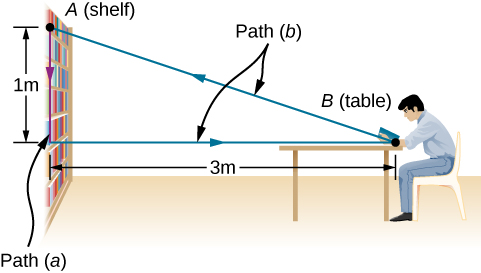Figure 7.5 Side view of the paths for moving a book to and from a shelf.

#### Strategy

We have just seen that the work done by a constant force of gravity depends only on the weight of the object moved and the difference in height for the path taken, ${W}_{AB}=\text{−}mg({y}_{B}-{y}_{A})$. We can evaluate the difference in height to answer (a) and (b).

#### Solution

1. Since the book starts on the shelf and is lifted down ${y}_{B}-{y}_{A}=\text{−}1\,\text{m}$, we have
$W=\text{−}(20\,\text{N})(-1\,\text{m})=20\,\text{J}\text{.}$
2. There is zero difference in height for any path that begins and ends at the same place on the shelf, so $W=0.$

#### Significance

Gravity does positive work (20 J) when the book moves down from the shelf. The gravitational force between two objects is an attractive force, which does positive work when the objects get closer together. Gravity does zero work (0 J) when the book moves horizontally from the shelf to the table and negative work (−20 J) when the book moves from the table back to the shelf. The total work done by gravity is zero $[20\,\text{J}+0\,\text{J}+(\text{−}20\,\text{J})=0].$ Unlike friction or other dissipative forces, described in Figure, the total work done against gravity, over any closed path, is zero. Positive work is done against gravity on the upward parts of a closed path, but an equal amount of negative work is done against gravity on the downward parts. In other words, work done against gravity, lifting an object up, is “given back” when the object comes back down. Forces like gravity (those that do zero work over any closed path) are classified as conservative forces and play an important role in physics.

Can Earth’s gravity ever be a constant force for all paths?

Show Solution

No, it’s only approximately constant near Earth’s surface.

### Work Done by Forces that Vary

In general, forces may vary in magnitude and direction at points in space, and paths between two points may be curved. The infinitesimal work done by a variable force can be expressed in terms of the components of the force and the displacement along the path,

$dW={F}_{x}dx+{F}_{y}dy+{F}_{z}dz.$

Here, the components of the force are functions of position along the path, and the displacements depend on the equations of the path. (Although we chose to illustrate dW in Cartesian coordinates, other coordinates are better suited to some situations.) Figure defines the total work as a line integral, or the limit of a sum of infinitesimal amounts of work. The physical concept of work is straightforward: you calculate the work for tiny displacements and add them up. Sometimes the mathematics can seem complicated, but the following example demonstrates how cleanly they can operate.

### Example

#### Work Done by a Variable Force over a Curved Path

An object moves along a parabolic path $y=(0.5\,{\text{m}}^{-1}){x}^{2}$ from the origin $A=(0,0)$ to the point $B=(2\,\text{m,}\,2\,\text{m})$ under the action of a force $\mathbf{\overset{\to }{F}}=(5\,\text{N/m})y\mathbf{\hat{i}}+(10\,\text{N/m})x\mathbf{\hat{j}}$ (Figure). Calculate the work done.

#### Strategy

The components of the force are given functions of x and y. We can use the equation of the path to express y and dy in terms of x and dx; namely,

$y=(0.5\,{\text{m}}^{-1}){x}^{2}\,\text{and}\,dy=2(0.5\,{\text{m}}^{-1})xdx.$

Then, the integral for the work is just a definite integral of a function of x.

#### Solution

The infinitesimal element of work is

$\begin{array}{cc}\hfill dW& ={F}_{x}dx+{F}_{y}dy=(5\,\text{N/m})ydx+(10\,\text{N/m})xdy\hfill \\ & =(5\,\text{N/m})(0.5\,{\text{m}}^{\text{−}1}){x}^{2}dx+(10\,\text{N/m})2(0.5\,{\text{m}}^{\text{−}1}){x}^{2}dx=(12.5\,{\text{N/m}}^{2}){x}^{2}dx.\hfill \end{array}$

The integral of ${x}^{2}$ is ${x}^{3}\text{/}3,$ so

$W={\int }_{0}^{2\,\text{m}}(12.5\,{\text{N/m}}^{2}){x}^{2}dx=(12.5\,{\text{N/m}}^{2}){\frac{{x}^{3}}{3}|}_{0}^{2\,\text{m}}=(12.5\,{\text{N/m}}^{2})(\frac{8}{3})=33.3\,\text{J}\text{.}$

#### Significance

This integral was not hard to do. You can follow the same steps, as in this example, to calculate line integrals representing work for more complicated forces and paths. In this example, everything was given in terms of x– and y-components, which are easiest to use in evaluating the work in this case. In other situations, magnitudes and angles might be easier.

Find the work done by the same force in Figure over a cubic path, $y=(0.25\,{\text{m}}^{\text{−2}}){x}^{3}$, between the same points $A=(0,0)$ and $B=(2\,\text{m,}\,2\,\text{m})\text{.}$

Show Solution

$W=35\,\text{J}$

You saw in Figure that to evaluate a line integral, you could reduce it to an integral over a single variable or parameter. Usually, there are several ways to do this, which may be more or less convenient, depending on the particular case. In Figure, we reduced the line integral to an integral over x, but we could equally well have chosen to reduce everything to a function of y. We didn’t do that because the functions in y involve the square root and fractional exponents, which may be less familiar, but for illustrative purposes, we do this now. Solving for x and dx, in terms of y, along the parabolic path, we get

$x=\sqrt{y\text{/}(0.5\,{\text{m}}^{-1})}=\sqrt{(2\,\text{m})y}\,\text{and}\,dx=\sqrt{(2\,\text{m})}\times \frac{1}{2}dy\text{/}\sqrt{y}=dy\text{/}\sqrt{(2\,{\text{m}}^{-1})y}.$

The components of the force, in terms of y, are

${F}_{x}=(5\,\text{N/m})y\,\text{and}\,{F}_{y}=(10\,\text{N/m})x=(10\,\text{N/m})\sqrt{(2\,\text{m})y},$

so the infinitesimal work element becomes

$\begin{array}{cc}\hfill dW& ={F}_{x}dx+{F}_{y}dy=\frac{(5\,\text{N/m})y\,dy}{\sqrt{(2\,{\text{m}}^{-1})y}}+(10\,\text{N/m})\sqrt{(2\,\text{m})y}\,dy\hfill \\ & =(5\,\text{N}\cdot {\text{m}}^{-1\text{/}2})(\frac{1}{\sqrt{2}}+2\sqrt{2})\sqrt{y}\,dy=(17.7\,\text{N}\cdot {\text{m}}^{-1\text{/}2}){y}^{1\text{/}2}dy.\hfill \end{array}$

The integral of ${y}^{1\text{/}2}$ is $\frac{2}{3}{y}^{3\text{/}2}$, so the work done from A to B is

$W={\int }_{0}^{2\,\text{m}}(17.7\,\text{N}\cdot {\text{m}}^{-1\text{/}2}){y}^{1\text{/}2}dy=(17.7\,\text{N}\cdot {\text{m}}^{-1\text{/}2})\frac{2}{3}{(2\,\text{m})}^{3\text{/}2}=33.3\,\text{J}\text{.}$

As expected, this is exactly the same result as before.

One very important and widely applicable variable force is the force exerted by a perfectly elastic spring, which satisfies Hooke’s law $\mathbf{\overset{\to }{F}}=\text{−}k\Delta \mathbf{\overset{\to }{x}},$ where k is the spring constant, and $\Delta \mathbf{\overset{\to }{x}}=\mathbf{\overset{\to }{x}}-{\mathbf{\overset{\to }{x}}}_{\text{eq}}$ is the displacement from the spring’s unstretched (equilibrium) position (Newton’s Laws of Motion). Note that the unstretched position is only the same as the equilibrium position if no other forces are acting (or, if they are, they cancel one another). Forces between molecules, or in any system undergoing small displacements from a stable equilibrium, behave approximately like a spring force.

To calculate the work done by a spring force, we can choose the x-axis along the length of the spring, in the direction of increasing length, as in Figure, with the origin at the equilibrium position ${x}_{\text{eq}}=0.$ (Then positive x corresponds to a stretch and negative x to a compression.) With this choice of coordinates, the spring force has only an x-component,${F}_{x}=\text{−}kx$, and the work done when x changes from ${x}_{A}$ to ${x}_{B}$ is

${W}_{\text{spring},AB}={\int }_{A}^{B}{F}_{x}dx=-k{\int }_{A}^{B}xdx=\text{−}k{\frac{{x}^{2}}{2}|}_{A}^{B}=-\frac{1}{2}k({x}_{B}^{2}-{x}_{A}^{2}).$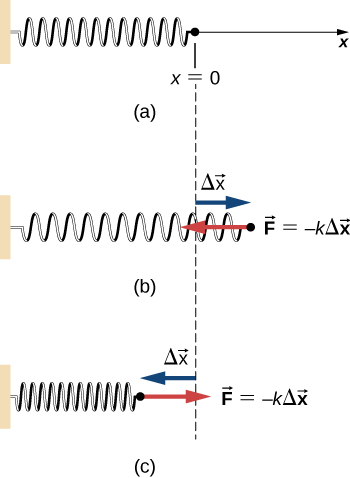Figure 7.7 (a) The spring exerts no force at its equilibrium position. The spring exerts a force in the opposite direction to (b) an extension or stretch, and (c) a compression.

Notice that ${W}_{AB}$ depends only on the starting and ending points, A and B, and is independent of the actual path between them, as long as it starts at A and ends at B. That is, the actual path could involve going back and forth before ending.

Another interesting thing to notice about Figure is that, for this one-dimensional case, you can readily see the correspondence between the work done by a force and the area under the curve of the force versus its displacement. Recall that, in general, a one-dimensional integral is the limit of the sum of infinitesimals,$f(x)dx$, representing the area of strips, as shown in Figure. In Figure, since $F=\text{−}kx$ is a straight line with slope $\text{−}k$, when plotted versus x, the “area” under the line is just an algebraic combination of triangular “areas,” where “areas” above the x-axis are positive and those below are negative, as shown in Figure. The magnitude of one of these “areas” is just one-half the triangle’s base, along the x-axis, times the triangle’s height, along the force axis. (There are quotation marks around “area” because this base-height product has the units of work, rather than square meters.)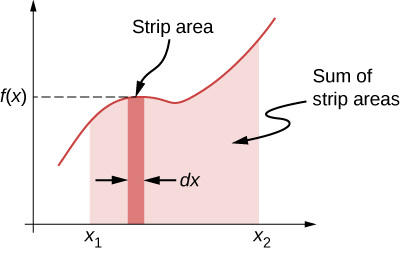Figure 7.8 A curve of f(x) versus x showing the area of an infinitesimal strip, f(x)dx, and the sum of such areas, which is the integral of f(x) from ${x}_{1}$ to ${x}_{2}$.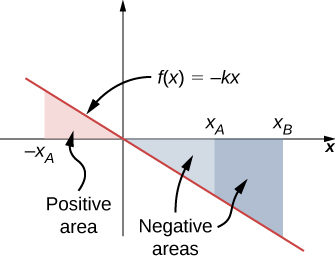Figure 7.9 Curve of the spring force $f(x)=\text{−}kx$ versus x, showing areas under the line, between ${x}_{A}$ and ${x}_{B}$, for both positive and negative values of ${x}_{A}$. When ${x}_{A}$ is negative, the total area under the curve for the integral in (Figure) is the sum of positive and negative triangular areas. When ${x}_{A}$ is positive, the total area under the curve is the difference between two negative triangles.

### Example

#### Work Done by a Spring Force

A perfectly elastic spring requires 0.54 J of work to stretch 6 cm from its equilibrium position, as in Figure(b). (a) What is its spring constant k? (b) How much work is required to stretch it an additional 6 cm?

#### Strategy

Work “required” means work done against the spring force, which is the negative of the work in Figure, that is

$W=\frac{1}{2}k({x}_{B}^{2}-{x}_{A}^{2}).$

For part (a), ${x}_{A}=0$ and ${x}_{B}=6\text{cm}$; for part (b), ${x}_{B}=6\text{cm}$ and ${x}_{B}=12\text{cm}$. In part (a), the work is given and you can solve for the spring constant; in part (b), you can use the value of k, from part (a), to solve for the work.

#### Solution

1. $W=0.54\,\text{J}=\frac{1}{2}k[{(6\,\text{cm})}^{2}-0]$, so $k=3\,\text{N/cm}\text{.}$
2. $W=\frac{1}{2}(3\,\text{N/cm})[{(12\,\text{cm})}^{2}-{(6\,\text{cm})}^{2}]=1.62\,\text{J}.$

#### Significance

Since the work done by a spring force is independent of the path, you only needed to calculate the difference in the quantity $½k{x}^{2}$ at the end points. Notice that the work required to stretch the spring from 0 to 12 cm is four times that required to stretch it from 0 to 6 cm, because that work depends on the square of the amount of stretch from equilibrium, $½k{x}^{2}$. In this circumstance, the work to stretch the spring from 0 to 12 cm is also equal to the work for a composite path from 0 to 6 cm followed by an additional stretch from 6 cm to 12 cm. Therefore, $4W(0\,\text{cm}\,\text{to}\,6\,\text{cm})=W(0\,\text{cm}\,\text{to}\,6\,\text{cm})+W(6\,\text{cm}\,\text{to}\,12\,\text{cm})$, or $W(6\,\text{cm}\,\text{to}\,12\,\text{cm})=3W(0\,\text{cm}\,\text{to}\,6\,\text{cm})$, as we found above.

The spring in Figure is compressed 6 cm from its equilibrium length. (a) Does the spring force do positive or negative work and (b) what is the magnitude?

Show Solution

a. The spring force is the opposite direction to a compression (as it is for an extension), so the work it does is negative. b. The work done depends on the square of the displacement, which is the same for $x=\pm6\,\text{cm}$, so the magnitude is 0.54 J.

### Summary

• The infinitesimal increment of work done by a force, acting over an infinitesimal displacement, is the dot product of the force and the displacement.
• The work done by a force, acting over a finite path, is the integral of the infinitesimal increments of work done along the path.
• The work done against a force is the negative of the work done by the force.
• The work done by a normal or frictional contact force must be determined in each particular case.
• The work done by the force of gravity, on an object near the surface of Earth, depends only on the weight of the object and the difference in height through which it moved.
• The work done by a spring force, acting from an initial position to a final position, depends only on the spring constant and the squares of those positions.

### Conceptual Questions

Give an example of something we think of as work in everyday circumstances that is not work in the scientific sense. Is energy transferred or changed in form in your example? If so, explain how this is accomplished without doing work.

Show Solution

When you push on the wall, this “feels” like work; however, there is no displacement so there is no physical work. Energy is consumed, but no energy is transferred.

Give an example of a situation in which there is a force and a displacement, but the force does no work. Explain why it does no work.

Describe a situation in which a force is exerted for a long time but does no work. Explain.

Show Solution

If you continue to push on a wall without breaking through the wall, you continue to exert a force with no displacement, so no work is done.

A body moves in a circle at constant speed. Does the centripetal force that accelerates the body do any work? Explain.

Suppose you throw a ball upward and catch it when it returns at the same height. How much work does the gravitational force do on the ball over its entire trip?

Show Solution

The total displacement of the ball is zero, so no work is done.

Why is it more difficult to do sit-ups while on a slant board than on a horizontal surface? (See below.)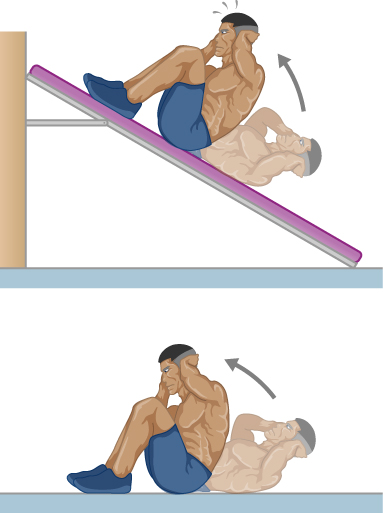As a young man, Tarzan climbed up a vine to reach his tree house. As he got older, he decided to build and use a staircase instead. Since the work of the gravitational force mg is path independent, what did the King of the Apes gain in using stairs?

Show Solution

Both require the same gravitational work, but the stairs allow Tarzan to take this work over a longer time interval and hence gradually exert his energy, rather than dramatically by climbing a vine.

### Problems

How much work does a supermarket checkout attendant do on a can of soup he pushes 0.600 m horizontally with a force of 5.00 N?

Show Solution

3.00 J

A 75.0-kg person climbs stairs, gaining 2.50 m in height. Find the work done to accomplish this task.

(a) Calculate the work done on a 1500-kg elevator car by its cable to lift it 40.0 m at constant speed, assuming friction averages 100 N. (b) What is the work done on the lift by the gravitational force in this process? (c) What is the total work done on the lift?

Show Solution

a. 593 kJ; b. –589 kJ; c. 0

Suppose a car travels 108 km at a speed of 30.0 m/s, and uses 2.0 gal of gasoline. Only 30% of the gasoline goes into useful work by the force that keeps the car moving at constant speed despite friction. (The energy content of gasoline is about 140 MJ/gal.) (a) What is the magnitude of the force exerted to keep the car moving at constant speed? (b) If the required force is directly proportional to speed, how many gallons will be used to drive 108 km at a speed of 28.0 m/s?

Calculate the work done by an 85.0-kg man who pushes a crate 4.00 m up along a ramp that makes an angle of $20.0^\circ$ with the horizontal (see below). He exerts a force of 500 N on the crate parallel to the ramp and moves at a constant speed. Be certain to include the work he does on the crate and on his body to get up the ramp.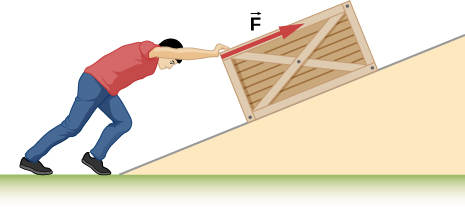Show Solution

3.14 kJ

How much work is done by the boy pulling his sister 30.0 m in a wagon as shown below? Assume no friction acts on the wagon.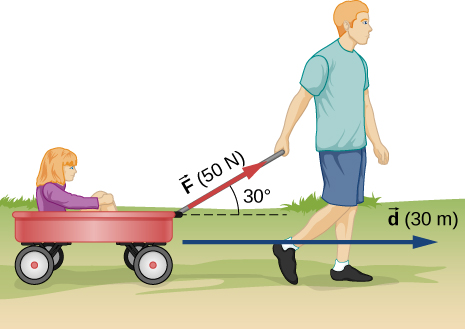A shopper pushes a grocery cart 20.0 m at constant speed on level ground, against a 35.0 N frictional force. He pushes in a direction $25.0^\circ$ below the horizontal. (a) What is the work done on the cart by friction? (b) What is the work done on the cart by the gravitational force? (c) What is the work done on the cart by the shopper? (d) Find the force the shopper exerts, using energy considerations. (e) What is the total work done on the cart?

Show Solution

a. –700 J; b. 0; c. 700 J; d. 38.6 N; e. 0

Suppose the ski patrol lowers a rescue sled and victim, having a total mass of 90.0 kg, down a $60.0^\circ$ slope at constant speed, as shown below. The coefficient of friction between the sled and the snow is 0.100. (a) How much work is done by friction as the sled moves 30.0 m along the hill? (b) How much work is done by the rope on the sled in this distance? (c) What is the work done by the gravitational force on the sled? (d) What is the total work done?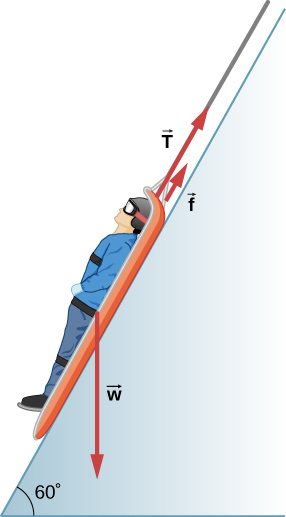A constant 20-N force pushes a small ball in the direction of the force over a distance of 5.0 m. What is the work done by the force?

Show Solution

100 J

A toy cart is pulled a distance of 6.0 m in a straight line across the floor. The force pulling the cart has a magnitude of 20 N and is directed at $37^\circ$ above the horizontal. What is the work done by this force?

A 5.0-kg box rests on a horizontal surface. The coefficient of kinetic friction between the box and surface is ${\mu }_{K}=0.50.$ A horizontal force pulls the box at constant velocity for 10 cm. Find the work done by (a) the applied horizontal force, (b) the frictional force, and (c) the net force.

Show Solution

a. 2.45 J; b. – 2.45 J; c. 0

A sled plus passenger with total mass 50 kg is pulled 20 m across the snow $({\mu }_{k}=0.20)$ at constant velocity by a force directed $25^\circ$ above the horizontal. Calculate (a) the work of the applied force, (b) the work of friction, and (c) the total work.

Suppose that the sled plus passenger of the preceding problem is pushed 20 m across the snow at constant velocity by a force directed $30^\circ$ below the horizontal. Calculate (a) the work of the applied force, (b) the work of friction, and (c) the total work.

Show Solution

a. 2.22 kJ; b. −2.22 kJ; c. 0

How much work does the force $F(x)=(-2.0\text{/}x)\,\text{N}$ do on a particle as it moves from $x=2.0\,\text{m}$ to $x=5.0\,\text{m?}$

How much work is done against the gravitational force on a 5.0-kg briefcase when it is carried from the ground floor to the roof of the Empire State Building, a vertical climb of 380 m?

Show Solution

18.6 kJ

It takes 500 J of work to compress a spring 10 cm. What is the force constant of the spring?

A bungee cord is essentially a very long rubber band that can stretch up to four times its unstretched length. However, its spring constant varies over its stretch [see Menz, P.G. “The Physics of Bungee Jumping.” The Physics Teacher (November 1993) 31: 483-487]. Take the length of the cord to be along the x-direction and define the stretch x as the length of the cord l minus its un-stretched length ${l}_{0};$ that is, $x=l-{l}_{0}$ (see below). Suppose a particular bungee cord has a spring constant, for $0\le x\le 4.88\,\text{m}$, of ${k}_{1}=204\,\text{N/m}$ and for $4.88\,\text{m}\le x$, of ${k}_{2}=111\,\text{N/m}\text{.}$ (Recall that the spring constant is the slope of the force F(x) versus its stretch x.) (a) What is the tension in the cord when the stretch is 16.7 m (the maximum desired for a given jump)? (b) How much work must be done against the elastic force of the bungee cord to stretch it 16.7 m?

Show Solution

a. 2.32 kN; b. 22.0 kJ

A bungee cord exerts a nonlinear elastic force of magnitude $F(x)={k}_{1}x+{k}_{2}{x}^{3},$ where x is the distance the cord is stretched, ${k}_{1}=204\,\text{N/m}$ and ${k}_{2}=-0.233{\,\text{N/m}}^{3}.$ How much work must be done on the cord to stretch it 16.7 m?

Engineers desire to model the magnitude of the elastic force of a bungee cord using the equation

$F(x)=a[\frac{x+9\,\text{m}}{9\,\text{m}}-{(\frac{9\,\text{m}}{x+9\,\text{m}})}^{2}]$,

where x is the stretch of the cord along its length and a is a constant. If it takes 22.0 kJ of work to stretch the cord by 16.7 m, determine the value of the constant a.

Show Solution

835 N

A particle moving in the xy-plane is subject to a force

$\mathbf{\overset{\to }{F}}(x,y)=(50\,\text{N}\cdot {\text{m}}^{2})\frac{(x\mathbf{\hat{i}}+y\mathbf{\hat{j}})}{{({x}^{2}+{y}^{2})}^{3\text{/}2}},$

where x and y are in meters. Calculate the work done on the particle by this force, as it moves in a straight line from the point (3 m, 4 m) to the point (8 m, 6 m).

A particle moves along a curved path $y(x)=(10\,\text{m})\left\{1+\text{cos}[(0.1{\,\text{m}}^{-1})x]\right\},$ from $x=0$ to $x=10\pi \,\text{m,}$ subject to a tangential force of variable magnitude $F(x)=(10\,\text{N})\text{sin}[(0.1{\,\text{m}}^{-1})x].$ How much work does the force do? (Hint: Consult a table of integrals or use a numerical integration program.)

Show Solution

257 J

### Glossary

work
done when a force acts on something that undergoes a displacement from one position to another
work done by a force
integral, from the initial position to the final position, of the dot product of the force and the infinitesimal displacement along the path over which the force acts